# Relative Strength Of Acids And Bases

## Relative Strength Of Acids And Bases

(i) Predict the relative acidic strength among the following

HF, HCl, HBr, HI.

Assume that all of these compounds have lost their proton.

So we get F , Cl , Br- , I

1. Volume available for the charge is increasing from left to right.

2. Charge density is decreasing from left to right.

3. Basic character of the ions is decreasing from left  to right.

4. Acidic character of the conjugate acids is increasing from left to right.

Therefore  increasing acidic strength is : HF <HCl <HBr <HI

(ii)  Predict the relative acidic strength among the following :

H2O, H2S,  H2Se,  H2Te.

Assume that all of these compounds have lost their proton.

So we get : HO, HS , HSe , HTe

It can be easily seen that the volume available for the negative charge is increasing from HO to HTe, therefore the following conclusion can be derived:

1. Volume available for the negative charge is increasing from left to right.

2. Charge density is decreasing from left to right.

3. Basic character is decreasing from left to right.

4. Acidic character of conjugate acids is increasing from left to right i.e.

H2O < H2S < H2Se < H2Te

(iii) Predict the relative acidic strength among the following:

CH3−H, H2N−H, HO−H, F−H

Assume that all of these compounds have lost their   protons. So we get CH3 , NH2, OH, F

We shall qualitatively analyse the charge density in each of the species and we shall follow the rule given below:

1. Larger the volume over which the charge is spread.

2. Smaller is the charge density.

3. Smaller is the basic character of the ion to attract a proton.

4. Larger is the acidic character of the conjugate acid.

In the above case one can see that, the size of the central atom over which the negative charge is present is decreasing from left to right. But it can also be seen that (3/4)th of the volume of C is  overlapped by hydrogen in CH3, 2/3 of the volume of N is overlapped by hydrogen in NH2, 1/2 the volume of oxygen is overlapped by hydrogen in OH where as, the entire volume of F is available for the charge in F. So the space available for the charge is increasing in the order CH3 < NH2 < OH < F. Therefore, the following conclusion can be derived:

1. Volume available for the negative charge is increasing in the conjugate bases from
left to right.

2. Charge density of the conjugate bases is decreasing from left to right.

3. Basic character of the conjugate bases is decreasing from left to right.

4. Acidic character of the acids is increasing from  left to right.

Therefore the increasing acidic character is CH4 < NH3 <H2O <HF.

(iv) Predict  the relative acidic strength among the following :

HOCl, HOClO, HOClO2 , HOClO3 .

Assume that all of these compounds have lost their proton.

So we get,OCl, OClO, OClO2, OClO3

The species that have more than one oxygen atoms show resonance.

Thus: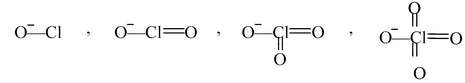It can be clearly seen that more the number of oxygen atoms, more the resonance and more is the volume available for the  negative charge. Therefore the following conclusion can be derived:

1. Volume available for the negative charge is increasing from left to right.

2. Charge density is decreasing from left to right.

3. Basic character of the anion is decreasing from left to right.

4. Acidic character of the conjugate acid is increasing from left to right.

Therefore, the order of acidic strength is:  HOCl < HOClO < HOClO2  < HOClO3

(v) In general, for oxo-acids with different central atoms of same oxidation states, acidic character  increases with the decrease in size of the central atom.

This is because, smaller the size of the central atom of an oxo- acid of the type HOY, larger is the electronegativity of Y, more is the pull of the electrons of O-Y bond towards  Y. This would result in a more positive character on O which would consequently help the H−O electrons to be pulled by O and release H as H+.

Therefore,

(a)  HOI < HOBr < HOCl (b)  HIO4 < HBrO4 < HClO4

(c)  HPO3 < HNO3 (c)  H3AsO4 < H3PO4.

For oxo-acids having the same central atom at different oxidation states, the rule to be followed is :

For oxo-acids of the general formulae, (HO)mZOn , where Z is the central atom, m is the number of oxygen atoms attached to Z and attached to H, while n is  the number of oxygen atoms attached to Z but not attached to H. It is these oxygen atoms which help in resonance and which determine the acidic strength.

For n= 0, acid is very weak e.g. HOCl, (HO)3Br

n = 1, acid is weak e.g. HOClO, HONO

n = 2, acid  is strong e.g. HOClO2, HONO2

n = 3, acid is very strong e.g. HOClO3, HOIO3

Acidic Strength of Organic Acids

The factors responsible for influencing the acidity of an organic compound, H−X are

(a) The strength of the H − X bond

(b) The electronegativity of X

(c) Factors stabilizing X as compared to HX.

(d) The nature of the solvent.

The most important factors among all is (c). Now let us see using any of these factors, how we predict the order of acidic strength for organic acids.

(i) Hydrocarbons: Among hydrocarbons, acidic strength increases with increase in the %
's' character. This is because, higher the 's' character, closer are the electrons to the nucleus of carbon and farther the electrons go from H. So H can be easily removed as H+.

Therefore, the order of acidic strength is CH3 - CH3 < CH2 = CH2 < CH ≡CH.

(ii) Alcohols: Alcohols are more acidic than CH4 because oxygen is more electro negative than C and consequently O − H bond easily give H+ than C - H bond.

Therefore, the order of acidic strength is H3C − H < CH3 − O − H.

If we were to predict the order of acidic strength of ethanol, isopropanol and tertiary butanol, we can proceed as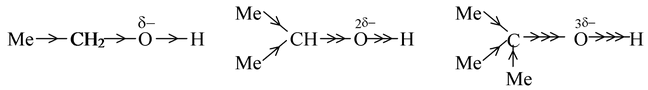As alkyl groups (methyl group) have a +I effect, the electron density at the oxygen atom will increase. More the number of alkyl groups, more will be the intensity of negative charge on oxygen. The greater the negative charge on the oxygen atom, the closer is the electron pair in the O−H bond driven to the hydrogen atom and consequently separation of a proton becomes increasingly difficult. Thus, the acidic strength of given alcohols will be in the order

Ethanol > isopropanol > t−butanol

(iii) Phenols and substituted phenols: Phenols are stronger acid than alcohols. This is attributed to the fact that in phenoxide (anion obtained by the loss of proton from phenol), there is possibility of the delocalization of its negative charge through interaction with the π−orbitals of the aromatic nucleus while alkoxide cannot be stabilized by such effect. Thus phenoxide is a weaker base than alkoxide. Consequently, the phenol would be stronger acid than alcohol.

PhOH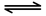PhO + H+

phenoxide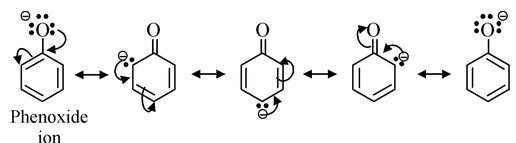Delocalization also occurs in the undissociated phenol but as it involves charge separation, it is less effective than in the phenoxide. Thus, phenoxide shows some reluctance to take up proton and the equilibrium lies to the right, indicating greater stabilization of phenoxide and more acidity of phenols with respect to alcohols.

Phenols are less acidic than carboxylic acids due to the fact that delocalozation of the negative charge in the carboxylate anion involves structures of identical energy content (as the negative charge resides on more electronegative atom, oxygen) while in phenoxide, the negative charge also resides on the less electronegative atom (carbon) making these structures of high energy content with respect to those structures in which the negative charge is on oxygen. Thus, the relative stabilization of the carboxylate with respect to the undissociated carboxylic acid is more effective than the relative stabilization of phenoxide w.r.t. to undissociated phenol. Thus, carboxylate is a weaker base than phenoxide, making the corresponding carboxylic acid, a stronger acid than phenol.

R−CO2HRCO-2 + H+

carboxylate ion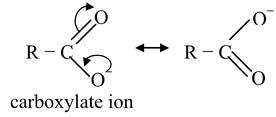The presence of electron−with drawing groups in phenol increases its acidity while the presence of electron releasing groups decreases the acidity of phenol.

Now, let us compare the acidic strength of o−, m− and p− nitrophenols. The corresponding phenoxide ions obtained from the three nitrophenols would be stabilized by
delocalization as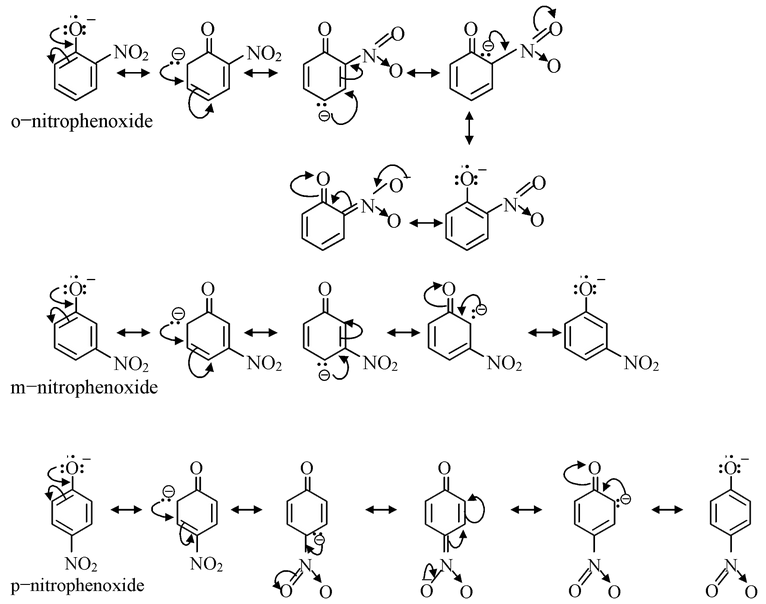From the structures of phenoxide ions, it is evident that o−and p−nitrophenoxides are more extensively stabilized with respect to m−nitro phenoxide (as additional contributing structure is obtained by the dispersal of negative charge over oxygen atom of NO2 group also in o− and p−nitrophenoxide but not in m−nitrophenoxide). Thus corresponding o− and p−nitrophenols are much stronger acids than m−nitrophenol. Out of o− and p−nitrophenols, p−nitrophenol is slightly stronger than o−isomer as o−isomer is bit stabilized by intramolecular hydrogen bonding, thus having a decreased tendency to release proton.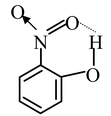The order of acidic strength of the nitrophenols is

p−nitrophenol > o−nitrophenol > m−nitrophenol

(iv) Aliphatic carboxylic acids: The aliphatic carboxylic acids are much stronger acids than phenols and alcohols. This is attributed to the fact that the carboxylate ion (obtained by the loss of proton from carboxylic acid) is relatively more stabilized by delocalization than the phenoxide and alkoxide ions with respect to the their undissociated molecules.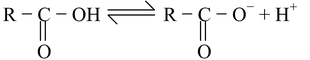The presence of electron−with drawing substituents in simple aliphatic acids increases their acidity while the electron−releasing substituents have reverse effect. For instance, let us compare the acidic strength of fluoroacetic acid and acetic acid.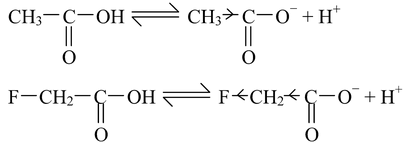The fluoro acetate ion is stabilized more due to strong −I effect of fluorine, with respect to acetate ion. Hence, fluro acetate ion is less basic than acetate ion, thereby making fluoroacetic acid stronger than acetic acid.

If there is a doubly bonded carbon atom adjacent to the carboxyl group, the acid strength is increased. This will be evident if we compare the acid strength of propanoic acid and propenoic (acrylic) acid.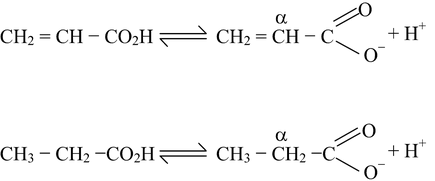As we know that unsaturated α−carbon atom in propionate ion is sp2 hybridised, which means that electrons are drawn closer to the carbon nucleus than in a saturated, sp3 hybridised α−carbon atom, due to greater s−contribution in the sp2 hybrid orbital. The result is that sp2 hybridised carbon atoms are less electron−donating than saturated sp3 hybridised ones. thus, propanoate ion will be comparatively less stabilized than prepenoate ion, thereby making propenoic acid stronger than propanoic acid (but propenoic will still be weaker than methanoic acid).

If there is a triple bonded carbon atom adjacent to carboxyl group, the acid strength is more with respect to the presence of a doubly bonded carbon atom adjacent to −CO2H group. This is attributed to the fact that sp hybridized carbon atoms are less electron−releasing than sp2 hybridized carbon atoms.

We can explain very strong acidic character of maleic acid than fumaric acid. If one proton is removed from each of the acids, the corresponding ions are formed. The maleate ion can be stabilized by chelation because hydrogen and oxygen are very near to each other, on the other hand fumarate ion can not be stabilized by chelation because hydrogen and oxygen are on the opposite sides, hence the formation of fumarate ion does not take place. This explains why maleic acid is a stronger acid than its isomer fumaric acid.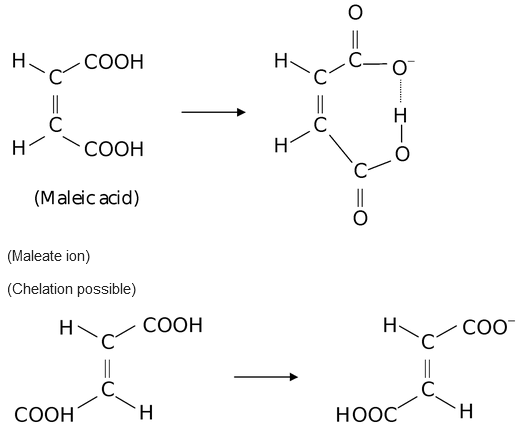(Fumaric acid) Fumarate ion (Chelation not possible)

(v) Aromatic carboxylic acids: Benzoic acid is a stronger acid than its saturated analogue cyclohexane carboxylic acid. This is due to the fact that phenyl group is less electron−donating (like a double bond) as compared to saturated carbon atom towards the carboxyl group, as the carbon atom to which −CO2H group is attached is sp2 hybridised in benzoic acid.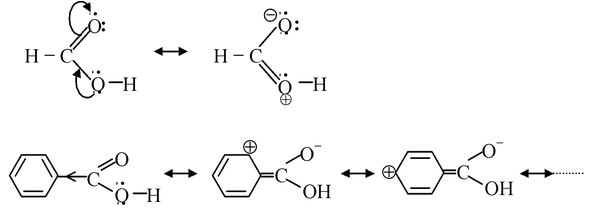As it is evident from the above structures that the lone pair on O atom of the OH group in formic acid undergoes delocalization with the CO group, thereby generating a positive charge centre on O atom which destabilize the O−H bond by withdrawing electron density from it. This consequently result in easy release of proton. While in benzoic acid, due to +R effect of phenyl group, the lone pair in O atom of the O−H group is prevented from entering into resonance with CO group to a large extent. This result in a smaller (partial) positive charge on the O atom of the O−H group in benzoic acid with respect to formic acid (the O atom of O−H group has full−fledge positive charge), so the release of proton becomes difficult in benzoic acid than formic acid.

Now let us consider substituted benzoic acids. At this point, we will consider the effect of substituents from m− and p−position only. First let us take methyl substituted benzoic acids. As the methyl group has +I effect, so the net result will be to increase the +R effect in the m− or p−tolyl group. Since, the inductive effect decreases with distance, so the +I effect of CH3 from para position would be less than the +I effect of CH3 group from meta position. Thus, the electron−density in the O−H bond of m−methyl benzoic acid would be greater than that in p−methyl benzoic acid. Accordingly, the p− isomer should be more acidic than the m−isomer. But in real practise, the order is reverse. Actually, we have ignored the hyperconjugation effect of CH3 group. Due to hyperconjugation effect of CH3 in p−isomer, the electron density in the O−H bond would be increased greatly with respect to that in m−isomer.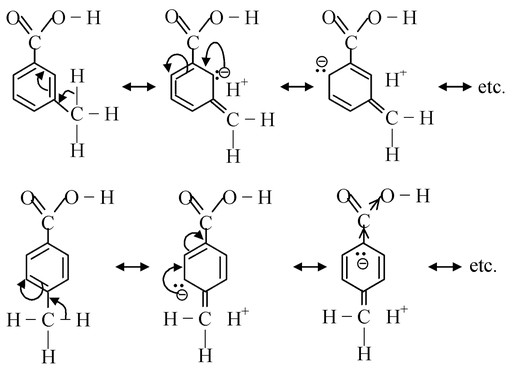Thus, the release of proton fascilitated easily in m−isomer than in p−isomer.

Secondly, let us take nitro substituted benzoic acids. As the −NO2 group exerts −I and −R effect (both reinforming each other), the electron−density from the O−H bond would be withdrawn, thus destabilizing the O−H bond and making m− and p− nitro benzoic acids stronger than benzoic acid.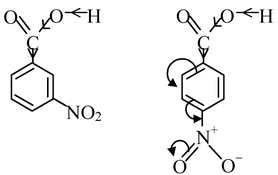As it is clear that −NO2 group exerts its electron withdrawing resonance effect from the p−position and not from m−position, thus withdrawal of electron density from the O−H bond is greater in p−nitro benzoic acid than m−nitro benzoic acid. Thus, p−isomer is stronger acid than m−nitro benzoic acid.

Thirdly, we compare the acid strength of m− and p−methoxy benzoic acid. OMe group exerts −I and +R effects. The electron−releasing resonance effect (+R) is operative in p−methoxy benzoic acid and not in m−methoxy benzoic acid while −I effect is operative in both the isomers. Thus, the electron density is greatly increased in the O−H bond in p−isomer than in m−isomer, thereby making m−isomer stronger acid than p−isomer.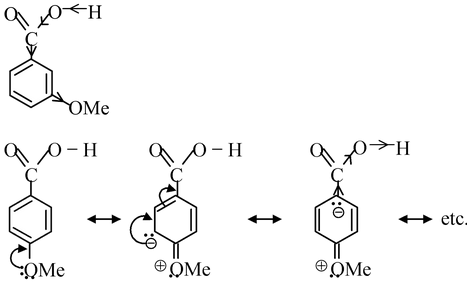There is a special effect shown by o−substituents called ortho−effect. It has been observed that irrespective of the nature of the group present at ortho position in benzoic acid, nearly all o−substituted benzoic acids are stronger than benzoic acid. As we have seen benzoic acid is a resonance hybrid and so the carboxyl group is coplanar with the ring. Introduction of any o−substituent tends to prevent this coplanarity. Thus, resonance is diminished (or prevented) and so the oxygen atom of the OH group has a greater positive charge, resulting in increased acid strength. Thus, it follows that greater the steric inhibition of resonance, the stronger is the acid.

o−hydroxy benzoic acid (salicylic acid) is far stronger than the corresponding m− and p−isomers. Steric inhibition of resonance can not explain this very large increase, since the corresponding methoxy benzoic acids all have similar strengths. The explanation here is the presence of intra molecular hydrogen bonding. Hydrogen of the −OH group can form a hydrogen bond with the carboxyl group whereas the Me of OMe cannot. Thus the carboxylate ion of o−hydroxy benzoic acid is stabilized by intramolecular hydrogen bonding while that of m−and p−isomer is not, making o−hydroxy benzoic acid stronger than the corresponding m− and p− isomers.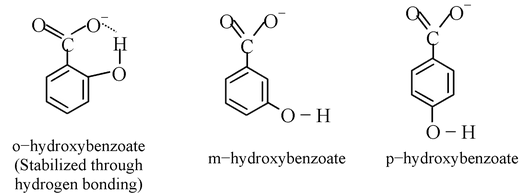Basic Strength of Organic Bases

Strength of bases is related to the ease of accepting a proton which inturn depends on the availability of electron pair on the nitrogen atom (or some other basic atom). More is the availability of electron pair, more easily the proton will be accepted and more will be the basic strength.

(i) Aliphatic Bases

As increasing strength in nitrogenous bases is related to the readiness with which they take up protons and which inturn depends on the availability of the unshared electron pair on nitrogen, we expect an increase in basic strength on going from NH3 → RNH2 → R2NH → R3N, due to the increasing inductive effect of successive alkyl groups making the nitrogen atom more electron rich.

But in actual practice the order of basic strength of amines is

NH3     < Me3N   < MeNH2 <  Me2NH

pKa's 9.25      9.80 10.64     10.77

NH3     < EtNH2   < Et3N    < Et2NH

and 9.25      10.67       10.88  10.93

It can be seen that the introduction of an alkyl group into ammonia increases the basic strength markedly as expected. The introduction of a second alkyl group further increases the basic strength, but the net effect of introducing the second alkyl group is very much less marked than with the first. However, the introduction of a third alkyl group to yield a tertiary amine, actually decreases the basic strength. This is due to the fact that the basic strength of an amine in water is determined not only by electron−availability on the nitrogen atom, but also by the extent to which the cation, (formed by the uptake of a proton), can undergo solvation and so become stabilized. The more hydrogen atoms attached to nitrogen in the cation, the greater the possibilities of extensive solvation via hydrogen bonding between protonated amines and water.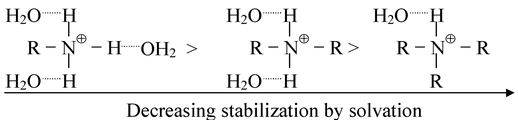Thus on going along the series, NH3 RNH2 R2NH R3N, the inductive effect will tend to increase the basicity but progressively less stabilization of the cation by hydration will tend to decrease the basicity. The net effect of introducing successive alkyl groups thus becomes progressively smaller, and an actual changeover takes place on going from a secondary to a tertiary amine. If this is assumed to be the right explanation, no such changeover should be observed if measurements of basicity are made in a solvent in which hydrogen−bonding cannot take place. This is indeed found to be true. In chlorobenzene, the order of basicity of the butylamines is BuNH2 < Bu2NH < Bu3N while their basic strength in water follows the order Bu3N  < BuNH2  < Bu2NH.

Tetraalkylammonium salts, e.g. R4N Ion treatment with moist silver oxide (AgOH) to yield basic solutions comparable in strength with the mineral alkalis. This is readily understandable as R4N OH formed is completely ionized to give R4N+ and free OH.

The effect of introducing electron−withdrawing groups, e.g. Cl, NO2, close to a basic centre decreases the basicity, due to their electron−withdrawing inductive effect. Thus the amine Tris (trifluoro methyl) amine is found to be virtually non−basic due to the presence of three powerfully electron−withdrawing CF3 groups.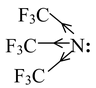The amides are also found to be only very weakly basic in water [pKa for ethanamide(acetamide) is ≈ 0.5], because of the −I and −R effect of RCO group which makes the electron pair very slightly available on nitrogen atom.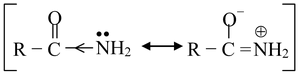If two C = O groups are present the resultant imides often become sufficiently acidic to form alkali metal salts. For example, benzene−1,2−dicarboximide is not basic but is acidic in nature because of the presence of two electron-withdrawing CO groups.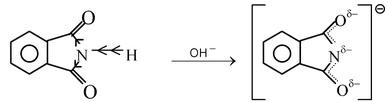(ii) Aromatic Bases

Aniline is a weaker base than ammonia or cyclohexylamine. It is because of the fact that the electron pair on nitrogen is involved in delocalization, making it less available for donation.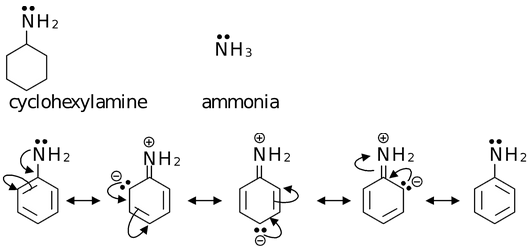If aniline accepts a proton, the anilinium cation formed cannot be stabilized by delocalization as the electron pair on nitrogen is no longer available. Thus, it is 'energitically unprofitable' for aniline to take up a proton. Thus it functions as a base with utmost reluctance.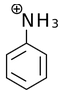Diphenylamine is even a weaker base than aniline due to the presence of another
phenyl group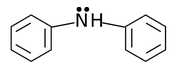and triphenylamine (Ph3N) is not basic at all by ordinary standards.

Introduction of alkyl group (like Me) on the nitrogen atom of aniline results in small increase in the basic strength.

C6H5NH2 < C6H5NHMe < C6H5NMe2

Unlike such introduction in aliphatic amines, this small increase in basic strength is progressive, indicating that cation stabilization through hydrogen−bonded solvation, here has less influence on the overall effect.

Let us compare the basic strengths of o−, m− and p−nitroanilines. The electron withdrawing NO2 group exerts its −R effect from o− and p−positions while its −I effect from all positions.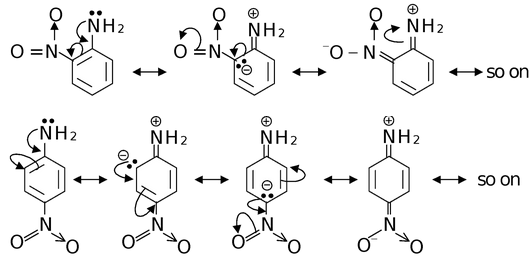Since, the electron pair on nitrogen in o−  and p−nitroanilines is delocalized extensively by −I and −R effect combindly, so they are less basic than m−nitroaniline in which only −I effect operates. Out of o− and p−isomers, ortho is less basic than para because −I effect of NO2 from ortho position is stronger than from para position. Thus the basic strength of nitro anilines follow the order

m−nitroaniline > p−nitroaniline > o−nitroaniline

Now if we take o−, m− and p−methoxy anilines. The OMe group exerts −I and +R effects (only from ortho and para position).

As OMe group exerts +R from ortho and para position, so o− and p−methoxy anilines are stronger base than m−methoxy aniline, in which OMe exerts only −I effect.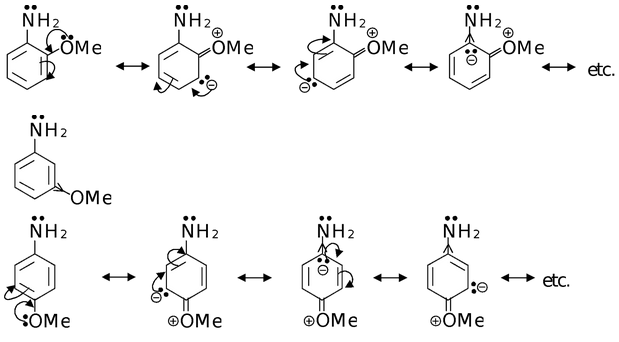−I effect of OMe from ortho position is stronger than from para position, thus decreasing the electron density on nitrogen in o−methoxy anilne than in p−methoxyaniline. Thus, the order of basic strength would be

p−methoxyaniline > o−methoxyaniline > m−methoxyaniline